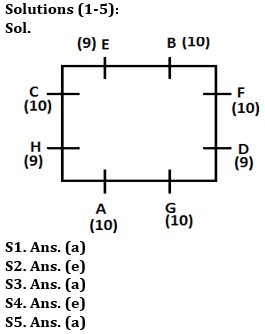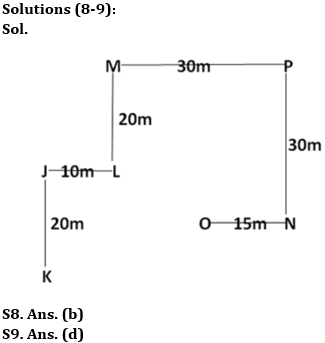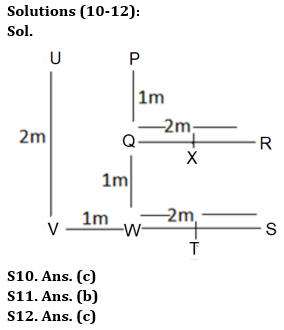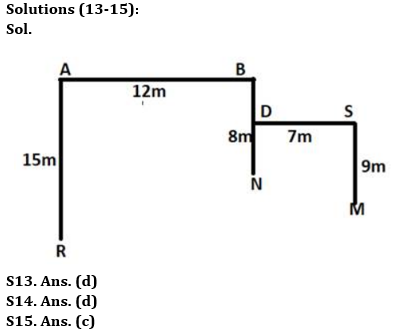Latest Banking jobs   »

# Reasoning Ability Quiz For IDBI AM/ Bank of India PO 2023 -10th April

Directions (1-5): Study the following information carefully and answer the questions given below:
Eight persons sit around a square table such that two persons sit on each side of the table (facing center). Each of them either have 9 or 10 toffees. B who has 10 toffees sits 4th left to A. None of the persons having 9 toffees sit together. Not more than one person sits between H and E. Not less than three persons have same number of toffees. F faces C and both have same toffees as A. Only one person sit between F and G. G is not neighbor of B. D has odd number of toffees and sits 2nd to the right of A. E is not 3rd right to A. Two persons sit between B and H.

Q1. Who among the following has 9 toffees?
(a) E
(b) F
(c) C
(d) B
(e) None of these

Q2. Who sit 4th left to the one who is immediate right to B?
(a) E
(b) F
(c) C
(d) B
(e) None of these

Q3. How many persons sit between D and E, when counted from right of D?
(a) Two
(b) One
(c) Three
(d) Four
(e) None of these

Q4. Who among the following sit immediate right to A?
(a) E
(b) F
(c) C
(d) B
(e) None of these

Q5. Four of the following belongs to a group find the one that does not belong to that group?
(a) E
(b) F
(c) C
(d) B
(e) G

Q6. In a certain code language, if ‘DEPLOYING’ is coded as ‘YPONLIGED’ and ‘OFFENSIVE’ is coded as ‘VSONIFFEE’, then what is the code for ‘LENDING’?
(a) NNLIGED
(b) NNLIEGD
(c) NNILGED
(d) NNLIGDE
(e) None of these

Q7. How many such pairs of letters are there in the word ‘QUADRATE’, each of which has as many letters between them in the word as in the English alphabet (Both forward and backward)?
(a) None
(b) One
(c) Two
(d) Three
(e) More than three

Directions (8-9): Study the following information carefully and answer the following questions.

Point N is 15m east of point O. Point P is 30m east of point M. Point J is 20m north of point K and 10m west of point L. Point N is 30m south of point P. Point L is 20m south of point M.

Q8. Point L is in which direction of point O?
(a) South
(b) North-west
(c) South-West
(d) North-East
(e) None of these

Q9. Point P is in which direction of point J?
(a) North
(b) East
(c) South-West
(d) North-East
(e) Can’t be determined

Directions (10-12): Study the following information carefully and answer the following questions.

P, Q, R, S, T, U, V, W and X are nine houses. R is 2m east of Q, which is 1m south of P, which is 2m north of W. V is 1m west of W while S is 3m east of V, which is 2m south of U. X is just in the middle of Q and R while T is just in the middle of W and S.

Q10. How far and in which direction is house U with respect to house P?
(a) 1m, East
(b) 2m, East
(c) 1m, West
(d) 2m, West
(e) 2m, North

Q11. House S is in which direction with respect to house R?
(a) North
(b) South
(c) South-East
(d) Can’t be determined
(e) None of these

Q12. Four of the following five are alike in a certain way and hence form a group. Which of the following pair does not belong to the group?
(a) QW
(b) VW
(c) QR
(d) TS
(e) PQ

Directions (13-15): Study the following information carefully and answer the following questions.
Point B is 8m to the north of Point N. Point A is 12m to the west of Point B. Point R is 15m to the south of Point A. Point S is 7m to the east of Point D which is mid-point of Point B and Point N. Point M is 9m to the south of Point S.

Q13. What is the total distance between Point D and Point M?
(a) 11m
(b) 9m
(c) 10m
(d) 16m
(e) None of these

Q14. What is the total distance between Point R and Point S?
(a) 42m
(b) 31m
(c) 39m
(d) 38m
(e) None of these

Q15. What is the direction of Point A with respect to Point M?
(a) South-east
(b) North-east
(c) North-west
(d) North
(e) None of these

SolutionsS6. Ans. (a)
Sol. In each word, all the letters are arranged in the descending order from left to right according to the alphabetical series. Therefore, option A is the correct answer.

S7. Ans. (c)
Sol. There are two pairs – RT, RU## FAQs

### What is the selection process of the IDBI AM?

The selection process of IDBI AM is Online exam and Interview.

#### Congratulations!Union Budget 2023-24: Free PDF